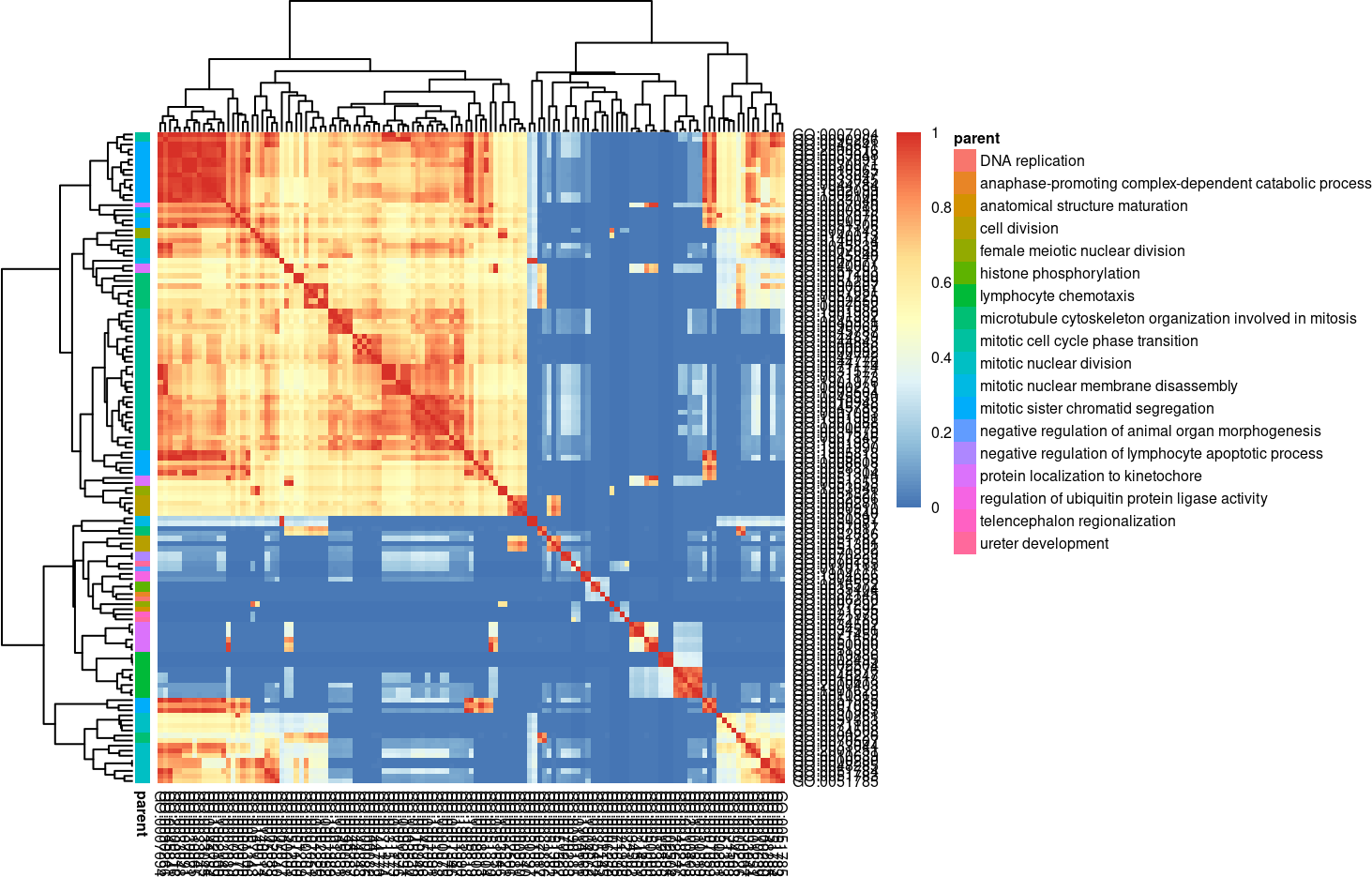# 1 Introduction to rrvgo

Gene Ontologies (GO) are often used to guide the interpretation of high-throughput omics experiments, with lists of differentially regulated genes being summarized into sets of genes with a common functional representation. Due to the hierachical nature of Gene Ontologies, the resulting lists of enriched sets are usually redundant and difficult to interpret.

`rrvgo` aims at simplifying the redundance of GO sets by grouping similar terms based on their semantic similarity. It also provides some plots to help with interpreting the summarized terms.

This software is heavily influenced by REVIGO. It mimics a good part of its core functionality, and even some of the outputs are similar. Without aims to compete, `rrvgo` tries to offer a programatic interface using available annotation databases and semantic similarity methods implemented in the Bioconductor project.

# 2 Using rrvgo

## 2.1 Getting started

Starting with a list of genes of interest (eg. coming from a differential expression analysis), apply any method for the identification of eneriched GO terms (see GOStats or GSEA).

`rrvgo` does not care about genes, but GO terms. The input is a vector of enriched GO terms, along with (recommended, but not mandatory) a vector of scores. If scores are not provided, `rrvgo` takes the GO term (set) size as a score, thus favoring broader terms.

## 2.2 Calculating the similarity matrix and reducing GO terms

First step is to get the similarity matrix between terms. The function `calculateSimMatrix` takes a list of GO terms for which the semantic simlarity is to be calculated, an `OrgDb` object for an organism, the ontology of interest and the method to calculate the similarity scores.

``````library(rrvgo)
simMatrix <- calculateSimMatrix(go_analysis\$ID,
orgdb="org.Hs.eg.db",
ont="BP",
method="Rel")``````

The `semdata` parameter (see `?calculateSimMatrix`) is not mandatory as it is calculated on demand. If the function needs to run several times with the same organism, it’s advisable to save the `GOSemSim::godata(orgdb, ont=ont)` object, in order to reuse it between calls and speedup the calculation of the similarity matrix.

From the similarity matrix one can group terms based on similarity. `rrvgo` provides the `reduceSimMatrix` function for that. It takes as arguments i) the similarity matrix, ii) an optional named vector of scores associated to each GO term, iii) a similarity threshold used for grouping terms, and iv) an orgdb object.

``````scores <- setNames(-log10(go_analysis\$qvalue), go_analysis\$ID)
reducedTerms <- reduceSimMatrix(simMatrix,
scores,
threshold=0.7,
orgdb="org.Hs.eg.db")``````

`reduceSimMatrix` selects as the group representative the term with the higher score within the group. In case the vector of scores is not available, `reduceSimMatrix` will get the GO term size from the `OrgDb` object and use it as the score, thus favoring broader terms. Please note that scores are interpreted in the direction that higher are better, therefore if you use p-values as scores, minus log-transform them before.

Higher thresholds force higher similarity between terms of a groups, resulting in more groups containing less similar terms.

## 2.3 Plotting and interpretation

`rrvgo` provides several methods for plotting and interpreting the results.

### 2.3.1 Similarity matrix heatmap

Plot similarity matrix as a heatmap, with clustering of columns of rows turned on by default (thus arranging together similar terms).

``````heatmapPlot(simMatrix,
reducedTerms,
annotateParent=TRUE,
annotationLabel="parentTerm",
fontsize=6)``````The function internally uses `pheatmap`, and further parameters can be passed to this function.

### 2.3.2 Scatter plot depicting groups and distance between terms

Plot GO terms as scattered points. Distances between points represent the similarity between terms, and axes are the first 2 components of applying a PCoA to the (di)similarity matrix. Size of the point represents the provided scores or, in its absence, the number of genes the GO term contains.

``scatterPlot(simMatrix, reducedTerms)``